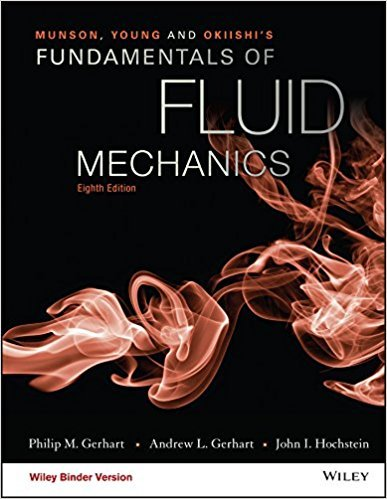×
Get Full Access to Fundamentals Of Fluid Mechanics - 8 Edition - Chapter 4.2.1 - Problem 4.55
Get Full Access to Fundamentals Of Fluid Mechanics - 8 Edition - Chapter 4.2.1 - Problem 4.55

×

# Assume the temperature of the exhaust in an exhaust pipecan be approximated by T = T0(1ISBN: 9781119080701 456

## Solution for problem 4.55 Chapter 4.2.1

Fundamentals of Fluid Mechanics | 8th Edition

• Textbook Solutions
• 2901 Step-by-step solutions solved by professors and subject experts
• Get 24/7 help from StudySoup virtual teaching assistantsFundamentals of Fluid Mechanics | 8th Edition

4 5 1 362 Reviews
26
3
Problem 4.55

Assume the temperature of the exhaust in an exhaust pipecan be approximated by T = T0(1 + aebx) [1 + c cos(t)], whereT0 = 100 C, a = 3, b = 0.03 m1, c = 0.05, and = 100 rad/s.If the exhaust speed is a constant 3 m/s, determine the time rate ofchange of temperature of the fluid particles at x = 0 and x = 4 mwhen t = 0.

Step-by-Step Solution:
Step 1 of 3

Lecture 5 notes Muscle System Muscle Properties o Excitability- respond to chemicals released from motor neurons o Conductivity- ability to propagate electrical signals over membrane o Contractility- ability to shorten and generate force o Extensibility- ability to be stretched without damaging tissue o Elasticity- ability to...

Step 2 of 3

Step 3 of 3

##### ISBN: 9781119080701

Since the solution to 4.55 from 4.2.1 chapter was answered, more than 215 students have viewed the full step-by-step answer. This full solution covers the following key subjects: . This expansive textbook survival guide covers 112 chapters, and 1357 solutions. This textbook survival guide was created for the textbook: Fundamentals of Fluid Mechanics, edition: 8. The answer to “Assume the temperature of the exhaust in an exhaust pipecan be approximated by T = T0(1 + aebx) [1 + c cos(t)], whereT0 = 100 C, a = 3, b = 0.03 m1, c = 0.05, and = 100 rad/s.If the exhaust speed is a constant 3 m/s, determine the time rate ofchange of temperature of the fluid particles at x = 0 and x = 4 mwhen t = 0.” is broken down into a number of easy to follow steps, and 71 words. Fundamentals of Fluid Mechanics was written by and is associated to the ISBN: 9781119080701. The full step-by-step solution to problem: 4.55 from chapter: 4.2.1 was answered by , our top Science solution expert on 03/16/18, 03:21PM.

Unlock Textbook Solution